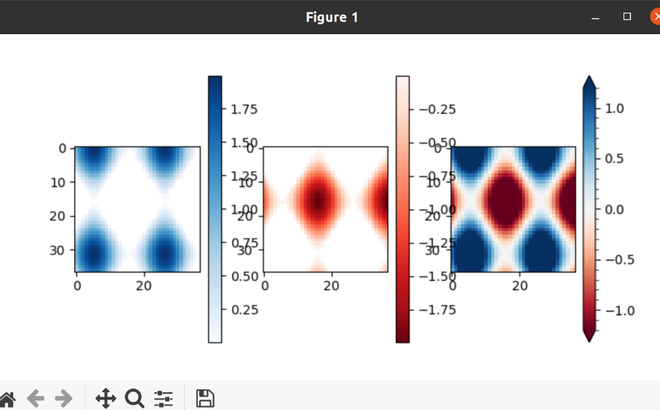GeeksforGeeks App
Open AppBrowser
Continue

# Set Colorbar Range in matplotlib

Hello Geeks! In this article, we will try to set the color range using the matplotlib Python module. Matplotlib allows us a large range of Colorbar customization. The Colorbar is simply an instance of plt.Axes. It provides a scale for number-to-color ratio based on the data in a graph. Setting a range limits the colors to a subsection, The Colorbar falsely conveys the information that the lower limit of the data is comparable to its upper limit. With the two different limits, you can control the range and legend of the Colorbar.

Requirement: Matplotlib, NumPy

For installation Matplotlib

`pip install matplotlib`

For installation Numpy.

`pip install numpy`

Let’s understand with step-wise implementation:

Step 1:

Import required library and set up some generic data.

## Python3

 `import` `numpy as np``import` `matplotlib.pyplot as plt`` ` `# setup some generic data``N ``=` `37``x, y ``=` `np.mgrid[:N, :N]``Z ``=` `(np.cos(x``*``0.2``) ``+` `np.sin(y``*``0.3``))`

Step 2:

Mask out the negative and positive values.

## Python3

 `Zpos ``=` `np.ma.masked_less(Z, ``0``)``Zneg ``=` `np.ma.masked_greater(Z, ``0``)`

Step 3:

Display data as an image, i.e., on a 2D regular raster.

## Python3

 `# plot just the positive data and save the``# color "mappable" object returned by ax1.imshow``pos ``=` `ax1.imshow(Zpos, cmap ``=` `'Blues'``, interpolation ``=` `'none'``)`

Step 4:

Plot both positive and negative values between +/- 1.2

## Python3

 `fig, (ax1, ax2, ax3) ``=` `plt.subplots(figsize``=``(``13``, ``3``), ``                                    ``ncols ``=` `3``)`` ` `pos_neg_clipped ``=` `ax3.imshow(Z, ``                             ``cmap ``=` `'RdBu'``, ``                             ``vmin ``=` `-``1.2``,``                             ``vmax ``=` `1.2``)``# Add minorticks on the colorbar to make ``# it easy to read the values off the colorbar.``color_bar ``=` `fig.colorbar(pos_neg_clipped, ``                         ``ax ``=` `ax3,``                         ``extend ``=` `'both'``)`` ` `color_bar.minorticks_on()``plt.show()`

Output:Below is the full implementation:

## Python3

 `import` `numpy as np``import` `matplotlib.pyplot as plt`` ` `# setup some generic data``N ``=` `37``x, y ``=` `np.mgrid[:N, :N]``Z ``=` `(np.cos(x``*``0.2``) ``+` `np.sin(y``*``0.3``))`` ` `# mask out the negative and positive values``Zpositive ``=` `np.ma.masked_less(Z, ``0``)``Znegative ``=` `np.ma.masked_greater(Z, ``0``)`` ` `fig, (ax1, ax2, ax3) ``=` `plt.subplots(figsize ``=` `(``13``, ``3``),``                                    ``ncols ``=` `3``)`` ` `# plot just the positive data and save the``# color "mappable" object returned by ax1.imshow``pos ``=` `ax1.imshow(Zpositive, cmap ``=` `'Blues'``)`` ` `# add the colorbar using the figure's method,``fig.colorbar(pos, ax ``=` `ax1)`` ` `# repeat everything above for the negative data``neg ``=` `ax2.imshow(Znegative, cmap ``=` `'Reds_r'``)``fig.colorbar(neg, ax ``=` `ax2)`` ` `# Plot both positive and negative values between +/- 1.2``pos_neg_clipped ``=` `ax3.imshow(Z, cmap ``=` `'RdBu'``,``                             ``vmin ``=` `-``1.2``, ``                             ``vmax ``=` `1.2``)`` ` `# Add minorticks on the colorbar to make ``# it easy to read the values off the colorbar.``color_bar ``=` `fig.colorbar(pos_neg_clipped, ``                         ``ax ``=` `ax3,``                         ``extend ``=` `'both'``)`` ` `color_bar.minorticks_on()``plt.show()`

Output:My Personal Notes arrow_drop_up